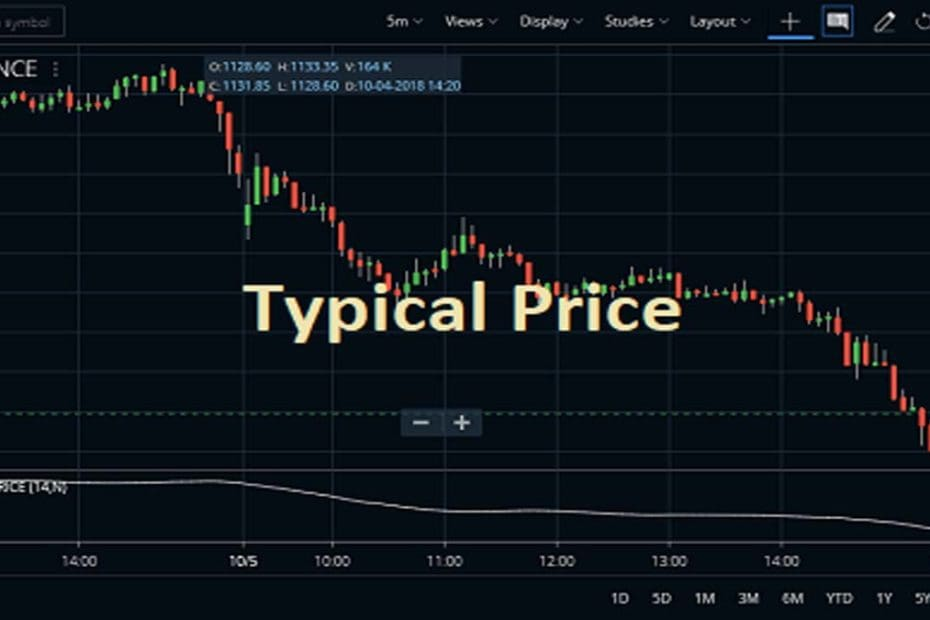Home » Blog » Trading » Technical Indicators » Typical Price Indicator Settings, Strategy, Formula

# Typical Price Indicator Settings, Strategy, Formula

• Technical IndicatorsThe Typical Price Indicator (TPI) function measures the average of the high, low, and closing prices for the day using a simple, single-line plot. The traders can use the Typical Price indicator for smoothing out some of the inconstancies of the closing price. The Typical Price Indicator is comprised of information for the whole trading day and not only the result of the end of the day.

## What is the Formula of the Typical Price Indicator?

The calculation of TPI is very easy and simple. In order to understand the outcome of TP, it is important to calculate the TPI over varying periods. This indicator uses three figures i.e. High Price, Low Price, and Closing Price. All of these High Price, Low Price, and Closing Price are added up and the outcome is divided by 3.

The formula of Typical Price = ( High Price + Low Price + Close Price ) / 3 or popularly known as HLC/3. This is also the same as the formula of the pivot point for any stock or index.

## How to Place the Typical Price Indicator in Charts?

The traders can know more about the TPI by attaching it to a chart. They can find it in the STUDIES section of Zerodha Kite. This indicator also available in Kite mobile App. The default Period is 14. If you want to change the color you can also do it from the parameters. The traders can also check the attachment process of the TPI in Reliance Industries share price chart. Here, you can attach any time limit chart including daily, weekly, monthly, hourly.

The next charting platform is Upstopx Pro. Here, after adding the script in the market watch, open any chart. Then search for the particular indicator and click on apply.

## What are the Usages of the Indicator?

• TPI is commonly used by traders to determine the support and resistance levels.
• This indicator determines the average figure of high, low and closing prices for the previous day.
• This indicator is a key component of the Money Flow Index.
• It can be used anywhere. The closing price is utilized to determine a trend as Typical Price (TP) uses the average price for a predetermined period and acts as a filter for Moving Average Systems.
• As Typical Price takes into account high, low and closing price, the indicator is preferred by traders instead of other closing price indicators.
• The indicator uses a single line plot to display the average value of Maximum, Minimum and Closing Price of a stock.
• The traders can compare the current average price with the previous ones to see if the market is bullish or bearish.

## Specifications of Typical Price Indicator :

The traders can use the TPI as the five-point system effectively. Traders must remind that Typical Price is the same as a short-term price predictor. This indicator cannot be used in the long run for determining price movements. It determines the market trend effectively. Another way, if the TPI is depicting an upward movement, then the market would be bullish. Also, if the TPI is illustrating a downward trend, then the market would be bearish.

## How Typical Price indicator works :

• The TPI gives a simple and single-line plot of the day’s average price. Some of the traders use the TPI rather than the closing price when creating moving-average penetration systems.
• In this indicator, if current TP is above previous TP then it is the bullish sentiment.
• Another way is if the previous TP outcome was greater than the current TP then the trend is bearish in the market.

Here in this above chart, I apply overlay Typical price indicator. As you can see when the price crosses the indicator from below and goes above it, I take the crossing bullish candles as buy signals. On the opposite side, when the indicator goes below the TPI line, sell signals generated.

The next strategy is when the TPI rises, the price rise, and once it falls the price also falls. So, it is a price following indicator.

Sometimes, you may see the divergence pattern. When the price and indicator movement does not match, divergence creates. Here also, in the picture below, the price made lower lows but the indicator made higher highs. So, it can assume that the price will move upward after a short period of time. And the exact thing happens here.

1. TPI can be used by any kind of trader likes Day Trader can determine TP of each day.
2. Until and unless a price breakout occurs the price trends are invariably following TPI.
3. This indicator can indicate New Top or Bottom in the market as well.
4. One of the famous system Five Point system calculates Typical Price, it greatly helps in determining the optimal time to enter a market or exit it.

1. TPI is an incomplete indicator. This requires the help of another technical indicator like MACD or Moving Average to determine future price trends.
2. This indicator doesn’t work well while signaling entry points. Traders are following the Typical Price indicator as it can reflect exit points only.

## Conclusion

So, the Typical Price indicator or TPI is basically an average of each day’s price. The Typical Price indicator gives a simple, single-line plot of each day’s average price. Many investors use the Typical Price rather than the closing price when creating an MA or moving average penetration system. We will come back with more useful indicator strategies later on our site. So stay updated for this.